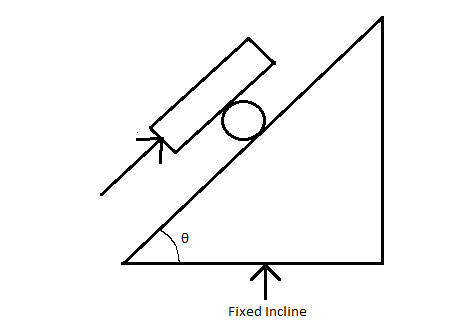# Just a classic Rotational Motion ProblemA solid cylinder of mass M and radius R is rolled up on an incline with the help of a plank of mass 2M as shown in the figure.A constant force F is acting on the plank parallel to incline. There is no slipping at any of the contact. All surfaces are rough. The force of friction between the plank and cylinder is given by

$\displaystyle{\frac { aF+bMgsin\theta }{ c } }$

Find least value of $a+b+c$

Details

$\bullet$ Here $a,b,c$ are positive integers.

×